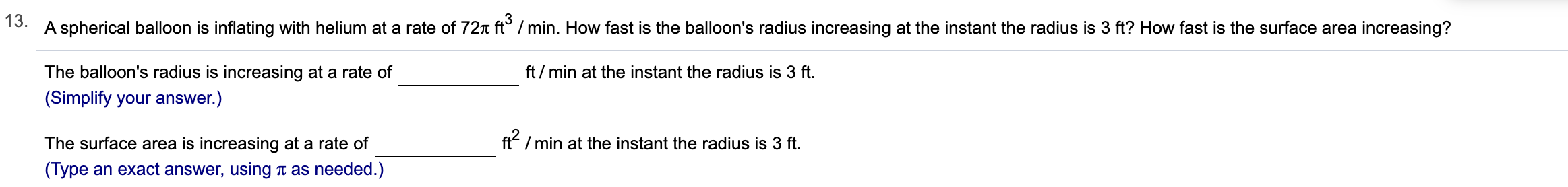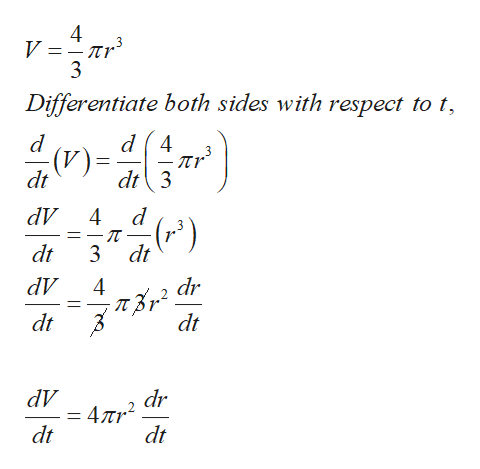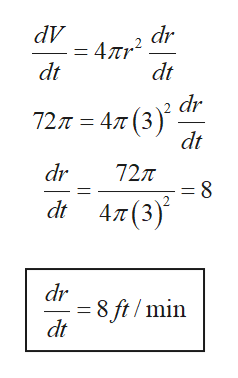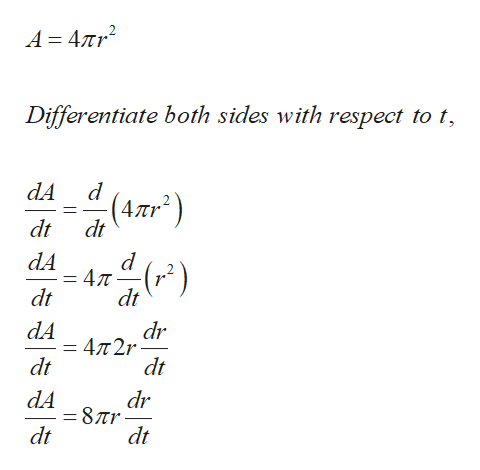# 13.A spherical balloon is inflating with helium at a rate of 72x ft° /min. How fast is the balloon's radius increasing at the instant the radius is 3 ft? How fast is the surface area increasing?The balloon's radius is increasing at a rate offt/min at the instant the radius is 3 ft.(Simplify your answer.)ft/min at the instant the radius is 3 ftThe surface area is increasing at a rate of(Type an exact answer, using i as needed.)

Question
334 views

Can you help me step by step?help_outlineImage Transcriptionclose13. A spherical balloon is inflating with helium at a rate of 72x ft° /min. How fast is the balloon's radius increasing at the instant the radius is 3 ft? How fast is the surface area increasing? The balloon's radius is increasing at a rate of ft/min at the instant the radius is 3 ft. (Simplify your answer.) ft/min at the instant the radius is 3 ft The surface area is increasing at a rate of (Type an exact answer, using i as needed.) fullscreen
check_circle

Step 1

The volume of a sphere is given as,help_outlineImage TranscriptioncloseV =-nr Differentiate both sides with respect to t d4 пr dt3 (V) dt dV (") dt dt dV 4 dr dt dt dV dr 4пr? dt dt fullscreen
Step 2

Given dv/dt=72ft3/min, r=3ft. Substitute the data in equation obtained in step1,help_outlineImage TranscriptionclosedV dr 4лr? dt dt dr 72л %3 4л (3) dt dr 727T = 8 4л(3)* dt dr = 8 ft /min dt fullscreen
Step 3

Surface area of a sphe...help_outlineImage TranscriptioncloseA = 47Tr2 Differentiate both sides with respect to t (4,mr) dt dt d dA dt dt () dA 47t2r dt dr dt dA = 877r- dt dr dt fullscreen

### Want to see the full answer?

See Solution

#### Want to see this answer and more?

Solutions are written by subject experts who are available 24/7. Questions are typically answered within 1 hour.*

See Solution
*Response times may vary by subject and question.
Tagged in

### Derivative